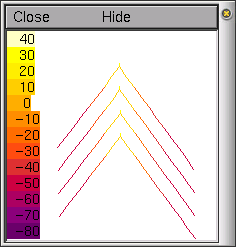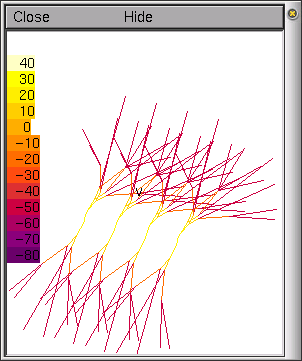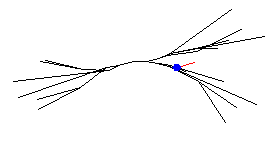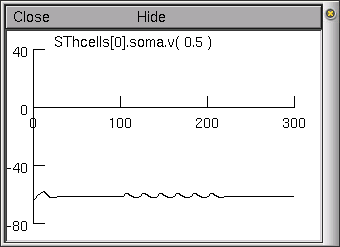# A NEURON Programming Tutorial - part C

## Introduction

At the beginning of part A, we defined our final product to be a model of a small network of rat subthalamic nucleus projection neurons with each neuron having a particular dendritic tree morphology.

In this part, we will explore how to introduce 3D spatial information into the model (i.e. where to place in 3D space dendrites and neurons), how to define multiple neurons using templates, and how to connect neurons up using NetCon.

## Templates

In part B, we created the first neuron that we need in our subthalamic network system. In this example we will create only 4 neurons, but we will create them in a way that increasing the number of neurons in our network is easy later on.

We need to copy the neuron we have already created to create additional neurons. NEURON provides us with a simple way to create multiple copies of the same neuron: templates.

A template is an object definition--it defines a prototype of an object from which we can create multiple copies. After defining the template, we must declare the object variable that we will use to reference the objects, just as we have in the past with the IClamp. Then, we can create a new instance of the object from the template that is an exact copy of the template. After we create the object from the template, we can either use it as it is or we can modify it to fit our needs. The structure of a template is as follows:

```begintemplate name
code
endtemplate name
```

where name is the name of the template we want to create, and code is any program commands that we want the template to include.

Here is a long definition of a template that illustrates several aspects of templates.

```begintemplate SThcell
public soma, dend

create soma, dend

proc init() {

ndend = 2

create soma, dend[ndend]

soma {
nseg = 1
diam = 18.8
L = 18.8
Ra = 123.0
insert hh
gnabar_hh=0.25
gl_hh = .0001666
el_hh = -60.0
}

dend {
nseg = 5
diam = 3.18
L = 701.9
Ra = 123
insert pas
g_pas = .0001666
e_pas = -60.0
}

dend {
nseg = 5
diam = 2.0
L = 549.1
Ra = 123
insert pas
g_pas = .0001666
e_pas = -60.0
}

// Connect things together
connect dend(0), soma(0)
connect dend(0), soma(1)
}
endtemplate SThcell
```

Creating new neurons from this template is straightforward. If we define an array of object variables:

```nSThcells = 4
objectvar SThcells[nSThcells]
```

we can then create all four of our cells using the new command in a for loop:

```for i = 0, nSThcells-1 {
SThcells[i] = new SThcell()
}
```

Each cell is a complete subthalamic neuron as we had described at the end of part B.

We will describe two main features of this template example:

• The public statement is used to tell NEURON what parts of the template can be accessed outside of the template definition. Normally, if there are no public names, then the code inside the template is completely private and nothing, aside from the name of the template itself, is accessible from the rest of the program code. For example, if we create a neuron template and we want to be able to put a current clamp in the soma of the neuron we create, we would need to give access to the soma section via the public command.

After declaring the neurons with the objectvar command and creating the objects with the new command, we can access a neuron soma using dot notation (e.g., SThcells.soma.L is the length of the soma in the 3rd neuron we created). In this template example both soma and dendrites are public allowing us to access them both through the dot notation. We can now insert current clamps into all of our neurons as follows:

```objectvar stim[nSThcells]

for i = 0, nSThcells-1 SThcells[i].soma {
stim[i] = new IClamp(0.5)
stim[i].del = 100
stim[i].dur = 100
stim[i].amp = 0.1
}
```
• The init() procedure. Most templates will have a special procedure called init() which is automatically called when a new object is created from the template. This is very useful to initialise the newly created object. In our init() procedure, above, we have created and defined all the sections in our neuron and appropriately connected them together. Thus when a neuron object is created from the template with the new command an entire subthalamic neuron is built.

Arguments to init()

Arguments can be passed to init() (passing arguments to procedures is described in part B) which can be used to affect the initialisation of the object via the parameters you pass to the new command. As an example of passing arguments to init() procedures, suppose we wanted to assess the differences in neurons with varying numbers of segments in their dendrites (nseg). We could do this in a single population of neurons creating multiple copies of neurons with different nseg values. If we write our init() procedure with an argument for nseg

```proc init() {

nsegdend = \$1
ndend = 2

create soma, dend[ndend]

...

dend {
nseg = nsegdend
diam = 3.18

...

dend {
nseg = nsegdend

...
}
```

Consequently, to create a neuron (say neuron 0) with dendritic sections containing, for example, 13 segments, we could create the neuron using:

```    SThcells = new SThcell(13)
```

Or to create all of our four cells with 3, 6, 9, and 12 dendritic segments respectively we could use the code

```for i = 0, nSThcells-1 {
SThcells[i] = new SThcell(3*(i+1))
}
```

Finally, we need to remember to set a default section so that graphing works:

```access SThcells.soma
```

## Positioning neurons in 3-DEach time we create a new section and connect it to others, NEURON places the section in a 3-D space and assigns an X, Y and Z coordinate to each end of the section. When creating more than one neuron, as we have above, each neuron is given a different Z coordinate for all of its sections. The X and Y coordinates of each neuron are determined by how the individual sections are connected. This makes viewing the neurons difficult since they are not arranged how we would normally think of them. To see the default position of neurons, open a space plot (under the Graph menu) and 3D rotate the neurons (see part B for instructions on space plots); an example is shown on the left.

Fortunately, NEURON provides a way to reposition each section in the 3-D space. We can use two functions to reposition each section: pt3dclear() and pt3dadd(). The first, pt3dclear(), will erase any 3-D positioning information associated with the section. The second, pt3dadd(), takes four arguments (X, Y, Z, and diam) and will add a new coordinate to the section. Usually there are coordinates for each end of the section which can be set by making two calls to pt3dadd()--once for the "0" end of the section and once for the "1" end of the section.

As you may be able to tell, there can be a lot of information to enter for each neuron, particularly for complex neuronal morphology where there are a large number of sections. There are a number of ways to set up this information. For example, neuron and section positions may be randomly placed using built in random distributions, or the tree portions may explicitly follow experimentally derived anatomical measurements, and these may be read from a file.

We will now dramatically enhance our neuron template, by reintroducing a more complete dendritic tree morphology read from two files (one for each of the two trees). The code for this is in the sthC2.hoc hoc file. The system is very similar to the neurons we have created so far (using the template defined above), except now, rather than two dendrites (each being a section) we have two trees, containing 23 and 11 dendritic branches respectively (these values are defined from the full tree morphology illustrated in part B). The tree properties are specified in the text files treeA.dat and treeB.dat. The files can have virtually any format, as how the information is read is specified in our template. These example files contain as their first line the number of sections in the tree. Each following line has the following format:

branch-num child1 child2 diam L X Y Z X Y Z

where branch-num is the reference number of the branch (starting at 1), child1 and child2 are the daughter branches reference numbers (0 if there is no daughter), diam and L are the branch diameter and length respectively, and the two 3D coordinate points are the branches 3D position (start and end points of the cylinder).

Our STh neuron template begins in a very similar manner to the example above:

```begintemplate SThcell
public soma, treeA, treeB

create soma, treeA, treeB
objectvar f

proc init() {local i, me, child1, child2

create soma

soma {
nseg = 1
diam = 18.8
L = 18.8
Ra = 123.0
insert hh
gnabar_hh=0.25
gl_hh = .0001666
el_hh = -60.0
}
```

We have made the soma, treeA and treeB public, so, for example, we could place electrodes anywhere along the dendritic trees. We have also created a new objectvar f used to reference the files. The soma definition is the same as we have previously used.

Note, we have not yet created our trees. Unlike the previous example, we no longer know the number of sections in the trees as this is now specified in the tree files (in their first lines).

However, notice that we have already created tree section arrays of length one just before the init() procedure. Because NEURON dynamically interprets the commands, each section and object variable must be declared before they are used. This means that in order for NEURON to know how to interpret commands about a section, it must be declared before the code that accesses it is interpreted by NEURON. For example, the following code would return an error since treeA is not created, in this example as an array of 2 sections, before the procedure is interpreted:

```begintemplate SThcell
public soma
create soma

proc init() {

create soma, treeA

treeA {
nseg = 5
diam = 3.18

...
```
Even though the create treeA command exists, treeA is not created until the procedure init is called. Thus, when NEURON interprets any treeA[i] code, it does not yet have any section of object named treeA, so it returns an error. To correct this we need to create the treeA and treeB sections outside of the init()procedure. Now we have a different problem: we do not know the correct number of treeA or treeB sections to create, since this information is read from the files. NEURON solves this problem by allowing you to redeclare a section or an array of sections inside a procedure. For this simple example, we need to create an array of treeA and treeB sections before the procedure init(). The number of elements in the array does not matter, since we will re-create the array inside the procedure.

In general, the two rules we need to follow are:

1. If a section or object is referenced inside a procedure, create or declare it before the procedure in which it is used.
2. When creating or declaring an array of sections or objects that will be recreated or redeclared inside a procedure, create or declare an array of length 1 before the procedure in which the sections or objects are recreated or redeclared.

To access a file, we need to create a new file object. This is done in a similar manner to creating other objects (for example the IClamp in part A):

```  f = new File()
f.ropen("treeA.dat")
```

The first line creates the file object, the second uses the file object function ropen() to open the file treeA.dat to read. To read a single value from the file we will use the function scanvar(). Thus we can read the correct number of sections in our tree from the first line of the file and then create them by redefining our tree sections:

```   ndendA = f.scanvar()
create treeA[ndendA]
```

Now we can continue to use f.scanvar() to read the rest of our file. For example, if the next line of our file treeA.dat was:

```  1  2  3  3.180 10.000 0.000 0.000 0.000 18.092 -0.346 4.932
```

then the second call to f.scanvar() returns the value 1, the third use of f.scanvar() returns the value 2, the fourth returns 3 and the fifth returns 3.180 etc. Thus we can define our dendritic tree treeA using the following code:

```    for i = 0,ndendA-1 {
me = f.scanvar() - 1
child1 = f.scanvar() - 1
child2 = f.scanvar() - 1

treeA[me] {
nseg = 1
diam = f.scanvar()
L = f.scanvar()
Ra = 123
// initialise and clear the 3D information
pt3dclear()
insert pas
g_pas = .0001666
e_pas = -60.0

if (child1 >= 0) {
connect treeA[child1](0), 1
}
if (child2 >= 0) {
connect treeA[child2](0), 1
}
}
}
```This is a loop creating each section/branch of the tree as defined by the file. The local variable me is the first value read from the file, and is the reference for this branch. As we know, arrays start at 0, however, our file references start at 1, so the variable me is defined as f.scanvar() - 1. Similarly the references for child1 and child2 branches have 1 subtracted. The branch diameter diam and length L are directly read from the file. Finally all the 3D position information is read from the file and the branch sections are connected up to form the tree.

The loop repeats this setup for each branch/section (note the number of tree sections for each tree is passed as the two parameters to the template). The second tree (treeB) is done in a very similar manner. To complete our template, after both trees have been read in from the files, we must connect the trees to the soma:

```    // Connect things to the soma
connect treeA(0), soma(1)
connect treeB(0), soma(0)
```

The final four neurons, each with a full dendritic tree morphology is shown here in a shape plot. This form of shape plot shows the voltage of the sections as a hot scale (hot colours mean high voltage, cool colours low voltage as indicated in the scale).

Note, in this example, each branch/section only has one segment, independent of how long the branch may be. You may want to increase the number of segments for a higher spatial resolution (see part B).

## Connecting the neurons together - NetCon

We have not yet connected our neurons together. Currently, they are operating as four independent subthalamic neurons, each with an electrode placed in the soma. The modern form of connecting neurons together is in an event based simulation model. Events are usually things like action potentials, as it is the events (rather than, for example, the specific voltage levels) that we need to pass or communicate between neurons. By using an event based model, we can dramatically reduce the amount of inter-neuron communication. This is important in simulation time optimisation and in simulations on parallel machines.

First we must add an additional public object variable to the neuron template. The new object variable will refer to a list that will hold an arbitrary number of NetCon objects. A NetCon object is an object associated with a particular source of events (usually a soma, where action potentials produce the binary events). So, we now begin our subthalamic neuron template with the following:

```begintemplate SThcell
public soma, treeA, treeB, nclist

create soma, treeA, treeB
objectvar f, nclist

proc init() {local i, me, child1, child2

create soma

nclist = new List()
```

The only thing we have changed is to add a object variable nclist, make it public, and in the init() procedure, associate it with a new List. In NEURON a List is an object that holds a list of other objects. The advantage of a list is that we don't have to specify in advance how big it will grow, as we do with an array. We will see later how to add items to a list and change their values.

Let's now modify the code so only one neuron is being stimulated by an electrode. Choose SThcells to have an electrode placed in the soma to produce action potentials between 100 and 200 ms. Having done Parts A and B of the tutorial, you should be able to modify the code and remove the electrodes from the other neurons.

Now, from 100 to 200 ms, neuron SThcells will be generating action potentials. In our first connection example, we only want to connect this neuron to SThcells and observe the EPSPs (Excitatory Post Synaptic Potentials) at the soma of neuron 0.

We must first create some synaptic objects. Like IClamps, discussed in part A, a synapse is simply an object that can be positioned anywhere on a neuron. For example, at the end of our current code we can define a new array of objects for our synapses. Here, we have a maximum of 10 synapses:

```maxsyn = 10
objectvar syn[maxsyn]
```

We will use the built in synaptic type ExpSyn, which is a synapse whose conductance instantaneously rises on receiving a spike and then exponentially decays. We position the synapse when it is created (in the same manner to IClamps) i.e. refer to a section of a neuron where we wish the synapse to be located and create the synapse there:

```SThcells.treeA syn = new ExpSyn(0)
```

In this example, we have created an ExpSyn synapse (syn) positioned at the proximal end of treeA dendritic branch 7 on subthalamic neuron 0 (marked here by the small blue square):Now we must define the source of events for this synapse. This will be the action potentials generated at the soma of SThcells. As SThcells receives the events (i.e. this is the neuron where the synapse is) we must append a new NetCon object to the nclist of this neuron. To create a new NetCon object, we use the command format:

```new NetCon(&source_v, synapse, threshold,
delay, weight)
```

where source_v is the source voltage (in our case the voltage from SThcells.soma), synapse is the object variable that refers to the synaptic object receiving the events (in our case syn), threshold is the threshold which the voltage must reach for it to be considered that an action potential has occurred, delay is the connection delay, and weight is the connection weight strength at the synapse. So to connect SThcells to the proximal end of treeA dendritic branch 7 on subthalamic neuron SThcells we add the command:

```SThcells.soma SThcells.nclist.append(new
NetCon(&v(1), syn, -20, 1, 0.5))
```

First this command accesses SThcells.soma, then the nclist of cell SThcells has a new NetCon object appended. This NetCon object has a source voltage of SThcells.soma.v(1) (note, as we have accessed SThcells.soma in the command above, we need only use the short variable v(1)). The NetCon object applies to syn which we have already attached to SThcells.treeA (see above). Our threshold for action potentials is -20mV, our delay 1ms, and our synaptic weight 0.5.

If we run the simulation and plot the voltage at SThcells.soma we see EPSPs resulting from the action potentials of neuron SThcells:## Dealing with lists

How do we change the weight, threshold and delay of our NetCon objects? If we had declared an objectvar called nc, we would have been able to refer to nc.weight, nc,threshold and nc.delay. However, our NetCon objects are embedded in a list, so we can't.

The solution is a group of commands to deal with lists. As mentioned above, the command

```nclist.append(obj)
```

appends the object specified by the object variable obj to the list nclist. We can use the command

```nclist.count()
```

to return the number of items in the list nclist. The command

```nclist.object(i)
```

returns the object at index i in the list nclist.

We can put these commands together to change properties of the connections. For example:

```for i = 0, SThcells.nclist.count()-1 {
SThcells.nclist.object(i).weight = 0.6
}
```

will change all of the weights onto SThcells to 0.6.

Try playing with the connection parameters (delay, threshold and weight) and then connect more of the neurons together using more of the synaptic objects (but remember we have only created 10 objectvars to refer to synaptic objects in this example; feel free to add more by increasing the maxsyn parameter).

In part D of this tutorial, we will make the neurons much more characteristic of subthalamic nucleus neurons by building our own channel types using NMODL.

Andrew Gillies (andrew@anc.ed.ac.uk)
David Sterratt (dcs@anc.ed.ac.uk)
based on the tutorials by Kevin E. Martin
with the assistance of Ted Carnevale and Michael Hines